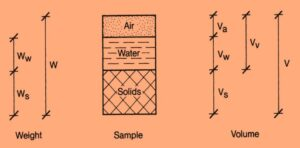# WEIGHT VOLUME RELATIONSHIP OF SOIL

The physical properties of a soil give insight as to the identification of the soil and the determination of its characteristics and load response. These properties can be determined by performing a laboratory analysis on undisturbed soil samples obtained during the test boring process.

The laboratory analysis should be performed in accordance with the following ASTM Standard:

D-854: Test Method for Specific Gravity of Soils

It should be noted that laboratory analyses are performed under controlled conditions with exacting materials and equipment. The results of such analyses may be considered to be accurate.

A field sample of undisturbed soil will contain three separate and distinct constituents – solids, water, and air.

One of the important properties that must be determined in the laboratory analysis is that of the weight-volume relationship of these constituents. The makeup of this soil sample can be illustrated visually as shown in Fig-1, where V represents volume, W represents weight, and the subscripts awand s represent air, water, and solids.The general procedure by which the weight and volume are determined is itemized below:

## STEP 1.

Select the sample to be tested and determine its total volume V. The units of volume are usually cubic centimeter (cm3).

## STEP 2.

Weigh the sample to determine its weight W, in g. Note that this weight includes both the weight of the water and the solid constituents.

## STEP 3.

The weight of the solid constituents w. must now be determined. The sample is first oven dried at a constant temperature of 105 to 115 degrees Centigrade. This will drive off all of the free water from the sample. If there are any clay particles in the sample the drying process will also remove the absorbed water molecularly bonded to those particles.

The sample which remains after the drying process consists solely of solid constituents, whose weight can now be determined.

## STEP 4.

The weight of water Ww originally contained in the sample can be determined by subtracting the weight of the solids from the initial weight of the sample:

Ww = W-Ws

## STEP 5.

The volume of water Vw, corresponding to the weight of water found in Step 3, may now be computed. Remember that density is the ratio of weight to volume, and that the density of water is 1 g/cm3; therefore:

Vw = Ww

## STEP 6.

The volume of solids v may be determined by placing the solids from Step 3 into a container of known volume, and filling the container with water whose volume is carefully measured. The difference between these two volumes represents the volume of the solids.

The weights and volumes obtained by these measurements can be used to determine important physical properties of the in-situ soil from which the undisturbed sample was obtained.

Open